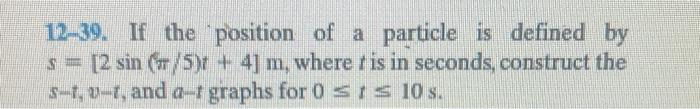Home / Expert Answers / Mechanical Engineering / 12-39-if-the-position-of-a-particle-is-defined-by-s-2-sin-pi-5-t-4-mathrm-m-where-pa234

# (Solved): 12-39. If the position of a particle is defined by $$s=[2 \sin (\pi / 5) t+4] \mathrm{m}$$, where ...12-39. If the position of a particle is defined by $$s=[2 \sin (\pi / 5) t+4] \mathrm{m}$$, where $$t$$ is in seconds, construct the $$s-t, v-t$$, and $$a-t$$ graphs for $$0 \leq t \leq 10 \mathrm{~s}$$.

We have an Answer from Expert